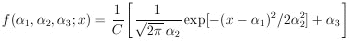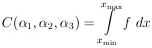### 9. SYSTEMATIC ERRORS

"Systematic effects" is a general category which includes effects such as background, selection bias, scanning efficiency, energy resolution, angle resolution, variation of counter efficiency with beam position and energy, dead time, etc. The uncertainty in the estimation of such a systematic effect is called a "systematic error". Often such systematic effects and their errors are estimated by separate experiments designed for that specific purpose. In general, the maximum-likelihood method can be used in such an experiment to determine the systematic effect and its error. Then the systematic effect and its error are folded into the distribution function of the main experiment. Ideally, the two experiments can be treated as one joint experiment with an added parameterM+1 to account for the systematic effect.

In some cases a systematic effect cannot be estimated apart from the main experiment. Example 2 can be made into such a case. Let us assume that among the beam of mono-energetic particles there is an unknown background of particles uniformly distributed in range. In this case the distribution function would bewhereThe solution3* is simply related to the percentage of background. The systematic error is obtained using Eq. 11.